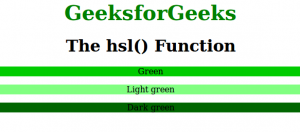# CSS | hsl() Function

• Last Updated : 31 Mar, 2021

The hsl() function is an inbuilt function in CSS which is used to define the colors using the Hue-saturation-lightness model (HSL).
Syntax:

`hsl( hue, saturation, lightness )`

Parameters: This function accepts three parameters as mentioned above and described below:

• hue: This parameter is used to define the degree on the color wheel. Its value lies between 0 to 360 where 0 or 360 represents red, 120 represents green and 240 represents blue.
• saturation: This parameter is used to define the saturation where 0% represents shade of gray and 100% represents full color.
• lightness: This parameter is used to define the lightness where 0% represents black, 50% represents normal, and 100% represents white.

Below program illustrates the hsl() function in CSS:
Program:

## html

 ```<``html``>``    ``<``head``>``        ``<``title``>hsl() function``        ``<``style``>``            ``.gfg1 {``                ``background-color:hsl(120, 100%, 40%);``                ``text-align:center;``            ``}``            ``.gfg2 {``                ``background-color:hsl(120, 100%, 75%);``                ``text-align:center``            ``}``            ``.gfg3 {``                ``background-color:hsl(120, 100%, 20%);``                ``text-align:center``            ``}``            ``.gfg {``                ``font-size:40px;``                ``font-weight:bold;``                ``color:green;``                ``text-align:center;``            ``}``            ``h1 {``                ``text-align:center;``            ``}``        ````    ````    ``<``body``>``        ``<``div` `class` `= ``"gfg"``>GeeksforGeeks``        ``<``h1``>The hsl() Function``        ``<``p` `class` `= ``"gfg1"``>Green` `        ``<``p` `class` `= ``"gfg2"``>Light green` `        ``<``p` `class` `= ``"gfg3"``>Dark green` `    `````

Output:Supported Browsers: The browser supported by hsl() function are listed below:

• Chrome 1.0 and above
• Internet Explorer 9.0 and above
• Firefox 1.0 and above
• Safari 3.1 and above
• Opera 9.5 and above

My Personal Notes arrow_drop_up